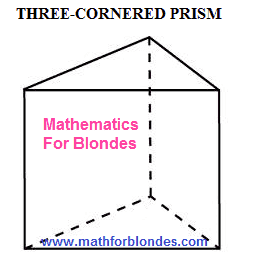## 4/13/2011

### How to calculate the area of surface of direct three-cornered prism

How to calculate the area of surface of direct three-cornered prism? For this purpose the best of all to imagine a three-cornered prism in all her beauty. How does a three-cornered prism look? Look at a pictureThree-cornered prism

Now next question: do you have a tube of lipstick in form three-cornered prism? If you answered this question (and or no) though as, means now you already know about what three-cornered prism and as she looks. Here those two triangles, from above and from below, are named the grounds of prism. Three rectangles on each side are named the verges of prism. As a triangle lies in founding of prism, verges for us exactly three. If there will be a pentagon in founding, verges it will be five. And if is there 1234-cornered in founding of prism? Correctly, verges there will be 1234 things. With the construction of direct prism we understood, after it it is possible to take up the mathematical calculations of area of surface of three-cornered prism.

As a prism is a geometrical body, her structure can be investigated on the example of body of blonde. That is included in the area of surface of prism? If you will come a heel on something sharp or knocked by the обо-что top of the blond head, it will be badly you. That is why both heels and top of head enter in the complement of surface of your body. Just overhead and lower triangles are included in the area of surface of three-cornered prism. If to dab with you a finger in a side, you will say "Ouch"! because a side belongs to your body. Here those three rectangles, that are from the sides of three-cornered prism, make the area of her side.

By the result of our scientific researches of bodies of blonde and three-cornered prism, we came to the conclusion, that the area of surface of three-cornered prism consists of areas overhead and lower grounds (they are equal) and area of side. The area of triangle needs to be found on one of formulas. I remind that the area of triangle found on a formula at the calculation of area of surface of prism needs to be taken two times, id est to increase her on two.

The area of side of prism is determined as a sum of areas of rectangular verges. It is needed to increase length of every side of triangle (that triangle that is in founding) on the height of prism and lay down three got areas together. It is possible to act simpler: to increase the perimeter of triangle (a perimeter of triangle is a sum of lengths of all his parties) on the height of prism. But adding up not to avoid you in any case: either to the increase (we fold long parties) or after an increase (we fold the areas of rectangular verges). Another trouble that you can lie in wait on a way to the area of surface of three-cornered prism is this absence of values of all lengths of parties. It is not mortal for the calculation of area of triangle. And for the area of side of prism you will have preliminary to find lengths of all three parties of triangle, applying the mathematical knowledge.